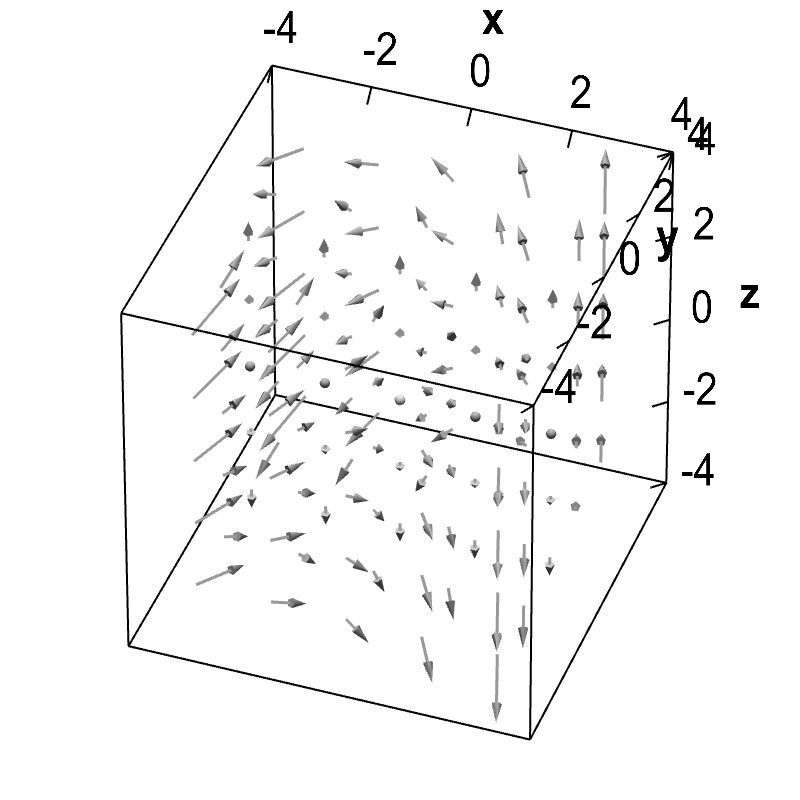# Math Insight

### Divergence and curl example

#### Formulas for divergence and curl

For $\dlvf: \R^3 \to \R^3$ (confused?), the formulas for the divergence and curl of a vector field are \begin{align*} \div \dlvf &= \pdiff{\dlvfc_1}{x} + \pdiff{\dlvfc_2}{y} + \pdiff{\dlvfc_3}{z}\\ \curl \dlvf &= \left(\pdiff{\dlvfc_3}{y}-\pdiff{\dlvfc_2}{z}, \pdiff{\dlvfc_1}{z} - \pdiff{\dlvfc_3}{x}, \pdiff{\dlvfc_2}{x} - \pdiff{\dlvfc_1}{y} \right). \end{align*} (The formula for curl was somewhat motivated in another page.) An alternative notation for divergence and curl may be easier to memorize than these formulas by themselves.

Given these formulas, there isn't a whole lot to computing the divergence and curl. Just “plug and chug,” as they say.

#### Example

Calculate the divergence and curl of $\dlvf = (-y, xy,z)$.

Solution: Since \begin{align*} \pdiff{\dlvfc_1}{x} = 0, \qquad \pdiff{\dlvfc_2}{y}=x, \qquad \pdiff{\dlvfc_3}{z}=1 \end{align*} we calculate that \begin{align*} \div\vc{F} = 0 + x + 1 = x+1. \end{align*} Since \begin{align*} \pdiff{\dlvfc_1}{y} = -1, \pdiff{\dlvfc_2}{x}=y,\\ \pdiff{\dlvfc_1}{z} =\pdiff{\dlvfc_2}{z} = \pdiff{\dlvfc_3}{x}=\pdiff{\dlvfc_3}{y}=0, \end{align*} we calculate that \begin{align*} \curl\dlvf = (0-0, 0-0, y+1) = (0,0,y+1). \end{align*}

Good things we can do this with math. If you can figure out the divergence or curl from the picture of the vector field (below), you doing better than I can.